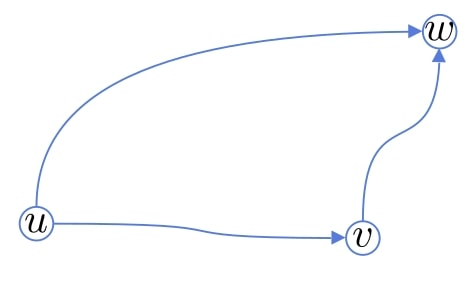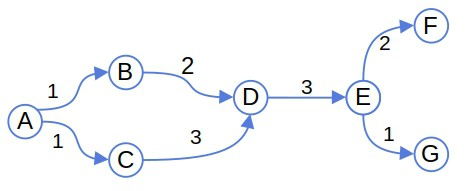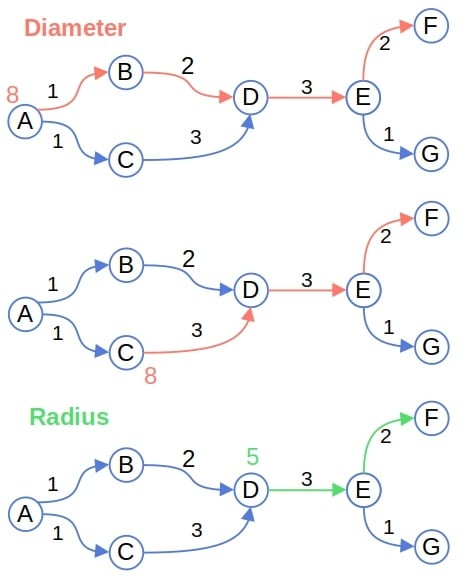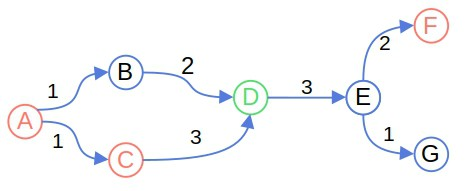## 1. Introduction

In this tutorial, we’ll explain five concepts from graph theory: eccentricity, radius, diameter, center, and periphery.

We’ll begin by defining the shortest path distance since the definitions of these concepts depend on it.

## 2. Graphs and Distances

Let’s say we have a graph, wheredenotes the nodes,the edges, andtheir weights (or lengths).

The lengthof the shortest simple path between any two nodesis a distance metric defined on the graph. Thus, the triangle inequality holds:That’s becauseis the length of the shortest path fromto, so any path between them is at least as long.

## 3. Eccentricity

The eccentricity of a node is the maximum of its distances to other nodes:For example, the eccentricity of the nodein the following graph is 8:This is the length of the path.

The diameter of a graph is the maximum eccentricity of its nodes:We define the radius as the minimum eccentricity:It’s worth noting that these two terms have multiple meanings. Diameters can also denote the longest paths in a graph, just as a radius can be any path whose length is equal to the graph’s minimum eccentricity.

In our example, the diameter is, and the radius is:### 4.1. The Relationship Between Radius and Diameter

We can prove that the diameter is bounded from above by twice the radius:This follows from the triangle inequality. Letandbe two distinct nodes whose distance is equal to the diameter:Letbe a central node of. The triangle inequality tells us that:Sinceis a central node, it holds that. Therefore:## 5. Center and Periphery

A node is peripheral if its eccentricity is equal to the graph’s diameter. All the nodes with that property constitute the graph’s periphery:On the other hand, the center of a graph is comprised of the nodes whose eccentricity is equal to the graph’s radius:In our example, the periphery contains,, and, while the only central node is:So, the peripheral nodes are at the opposite ends of the graph, while the central nodes are in between.

An example of when these concepts may be useful in determining the optimal placement of facilities on a graph. If we model a city map as a graph, it makes sense to build a facility at a central node because that will minimize the longest distance a citizen will have to travel to reach the facility.

Conversely, if the goal is to maximize the distance between two objects, we’d place them on the locations of two peripheral nodes on opposite sides of the town.